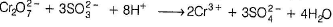Courses

# Test: General Chemical & Magnetic Properties Of d- And f-Block Elements

## 25 Questions MCQ Test Chemistry for JEE | Test: General Chemical & Magnetic Properties Of d- And f-Block Elements

Description
This mock test of Test: General Chemical & Magnetic Properties Of d- And f-Block Elements for JEE helps you for every JEE entrance exam. This contains 25 Multiple Choice Questions for JEE Test: General Chemical & Magnetic Properties Of d- And f-Block Elements (mcq) to study with solutions a complete question bank. The solved questions answers in this Test: General Chemical & Magnetic Properties Of d- And f-Block Elements quiz give you a good mix of easy questions and tough questions. JEE students definitely take this Test: General Chemical & Magnetic Properties Of d- And f-Block Elements exercise for a better result in the exam. You can find other Test: General Chemical & Magnetic Properties Of d- And f-Block Elements extra questions, long questions & short questions for JEE on EduRev as well by searching above.
QUESTION: 1

### Only One Option Correct Type Direction (Q. Nos. 1-10) This section contains 10 multiple choice questions. Each question has four choices (a), (b), (c) and (d), out of which ONLY ONE is correct. Q. Of the following outer electronic configurations of atoms, the highest oxidation state is achieved by which one of them?

Solution:

(n - 1) d5ns2 attains the maximum oxidation state of+ 7.

QUESTION: 2

### Among the following series of transition metal ions, the one where all metal ions have 3d2 electronic configuration is (Atomic number, Ti = 22, V = 23, Cr = 24, Mn = 25)  [AIEEE 2004]

Solution:

Ti2+,V3+,Cr4+ and Mn5+ show 1s22s22p63s23p64s03d2 electronic configuration.

QUESTION: 3

### For the four successive transition elements (Cr, Mn, Fe and Co), the stability of + 2 oxidation state will be there in which of the following order?

Solution:

Mn2+ (d5) > Cr2+ (d4) > Fe2+ (d6) > Co2+ (d7) Mn2+ is more stable due to half-filled configuration, i . e . d5.

QUESTION: 4

The catalytic activity of transition metals and their compounds is mainly due to

Solution:

The variability of oxidation state, a characteristic of transition element arises due to incomplete filling of d-orbitals.

QUESTION: 5

Many copper (I) compounds are unstable in aqueous solution and undergo disproportionation as 2Cu+ → Cu + Cu2+ . This is due to

Solution:

Cu (I) undergoes spontaneous disproportionation.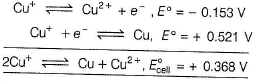hus, ΔG is negative (spontaneous) for disproportionation.

QUESTION: 6

Gun metal is an alloy of

Solution:

Gun metal is an alloy of Cu, Zn and Sn. It contains 88% Cu 10% Sn and 2% Zn.

QUESTION: 7

In which of the following pairs both the ions are coloured in aqueous solution? (Atomic number, Sc = 21, Ti = 22, Ni = 28, Cu = 29, Co = 27)

Solution:

Ni2+ and Ti3+ ions are coloured in aqueous solution because they contain unpaired electrons.

QUESTION: 8

[Ti (H2O)6]3+ absorbs green and yellow region part of visible light. Then the transmitted colour of the compound is

Solution:

We know that white light is composed of many different colours. When white light is allowed to fall on [Ti(H2O)6]3+ ion, this ion absorbs the light of green and yellow colour and transmits is almost purple colour.The purple colour of the transmitted light is called complimentary colour of that of absorbed light.

QUESTION: 9

Compound of metal ion MX+(Z = 26) has a spin only magnetic moment of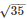BM The number of unpaired electrons and x value in the compound are

Solution:

Metal ion is Fe3+ which have 5 unpaired electrons.

QUESTION: 10

In which of the following the stability of two oxidation states is correctly represented?

Solution:

Mn2+ (d5) is more stable than Mn3+(d4) because Mn2+ have half-filled configuration.

*Multiple options can be correct
QUESTION: 11

One or More than One Options Correct Type

Direction (Q. Nos. 11-15) This section contains 5 multiple choice questions. Each question has four choices (a), (b), (c) and (d), out of which ONE or MORE THAN ONE are correct.

Q.

Which one of the following arrangements represent the correct order of the property stated against it?

Solution:

Due to the presence of unpaired electrons, transition elements are paramagnetic in nature. Mn2+ contains 5 unpaired electrons while Fe2+ contains 4 unpaired electrons.
Manganese exhibits all the oxidation states from + 2 to + 7.

*Multiple options can be correct
QUESTION: 12

The metals present in German silver alloy are

Solution:

It is a copper alloy with nickel and often zinc. The usual formation is 60% copper, 20% nickel and 20% zinc.

QUESTION: 13

Paramagnetic Curie temperature in Kelvin for iron is equal to :

Solution:

Paramagnetic Curie temperature for iron is equal to 1095 Kelvin. Relative permeability is greater than 1 for paramagnetic material.

*Multiple options can be correct
QUESTION: 14

+8 oxidation state is/are shown by

Solution:

+8 oxidation state shown by Ru and Os.

*Multiple options can be correct
QUESTION: 15

Ions having same colour in aqueous solution are

Solution:

Ni2+ and Fe2+ have green colour and V4+ and Cr2+ have blue colour.

QUESTION: 16

Comprehension Type

Direction (Q, Nos. 16 and 17) This section contains a paragraph, describing theory, experiments, data, etc. Two questions related to the paragraph have been given. Each question has only one correct answer among the four given options (a), (b), (c) and (d).

Passage

Transition metals and their compounds have paramagnetic properties due to the presence of unpaired electrons in (n - 1)d-orbitals. The paramagnetic behaviour is expressed in terms of magnetic moment which is because of the spin of the unpaired electrons (n). It is given as
Magnetic moment =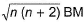Majority of transition metal compounds are coloured both in solid state as well as in aqueous solution. This is also due to the presence of unpaired electrons in (n - 1) d-orbitals, d-orbitals splitting and d-d transition of electrons absorbing suitable visible light.

Q.

Which of the following exhibit colour due to charge transfer phenomenon, but not due to d-d transition?

Solution:

In transition metal complexes, a change in electron distribution between the metal and a ligand give rise to charge transfer bonds.
Here, charge transfer may occur from the ligand molecular orbitals to the empty or partially filled metal d-orbitals.

QUESTION: 17

Transition metals and their compounds have paramagnetic properties due to the presence of unpaired electrons in (n - 1)d-orbitals. The paramagnetic behaviour is expressed in terms of magnetic moment which is because of the spin of the unpaired electrons (n). It is given as
Magnetic moment =Majority of transition metal compounds are coloured both in solid state as well as in aqueous solution. This is also due to the presence of unpaired electrons in (n - 1) d-orbitals, d-orbitals splitting and d-d transition of electrons absorbing suitable visible light.

Q.

Which pair of ions are expected to be diamagnetic?

Solution:

Ag+ (d10) and Au+(d10) are diamagnetic due to absence of unpaired number of electrons.

QUESTION: 18

Matching List Type

Direction (Q. No. 18-20) Choices fo r the correct combination of elements from Column I and Column II are given as options (a), (b), (c) and (d), out of which one is correct.

Q.

Match the Column I with Column II and mark the correct option from the given codes.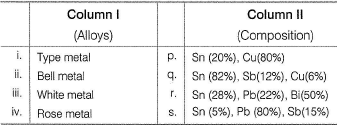Solution:

(i) → (s),(ii) → (p),(iii) → (q) (iv) → (r)

QUESTION: 19

Match the Column I with Column II and mark the correct option from the given codes.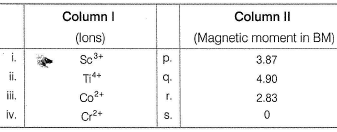Solution:

(i) → (s), (ii) → (s), (iii) → (p),(iv) → (q)

QUESTION: 20

Match the Column I with Column II and mark the correct option from the given codes.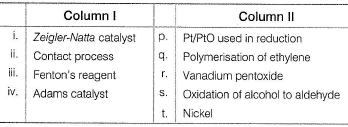Solution:

Fenton’s reagent (Fe SO4 + H2O2) is used for oxidising alcohol to aldehyde. Zeigler-Natta catalyst [AI(C2H5)3 + TiCI4] is used for manufacture of polythene.
(i) → (Q). (ii) → (r), (iii) → (s), (iv) → (p)

*Answer can only contain numeric values
QUESTION: 21

One Integer Value Correct Type

Direction (Q. Nos. 21-24) This section contains 4 questions. When worked out will result in an integer from 0 to 9 (both inclusive).

Q.

Number of alloys that contain nickel among the following solder, gun metal, German silver, nichrome, monel metal, constanton, bell metal, duralumin, type metal, invar, alnico.

Solution:

German silver (Cu + Zn + Ni), nichrome (Ni 60% + Cr 20% + Fe), monel metal (Cu + Ni 66%), constanton (Cu + Ni), invar (Fe + Ni), alnico (Al + Ni + Co + Cu).

*Answer can only contain numeric values
QUESTION: 22

Green vitriol is FeSO4.xH2O and white vitriol is ZnSO4.yH2O, Then, the values of x and y are

Solution:

In both, xand y value is 7.

*Answer can only contain numeric values
QUESTION: 23

Oxidation state of chromium in CrO5 is +x. Here, value of x is

Solution: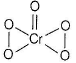4 oxygen atoms attached by peroxide linkage. So, their oxidation states are taken as -1.
Now, let the oxidation state of Cr be x.
then x + 4 x (-1) - 2 = 0
x = 6

*Answer can only contain numeric values
QUESTION: 24

Number of compounds in which metal has zero oxidation state

WO3,Ni(CO)4, MoO3, Fe(CO)5, Cr(CO)6, [Pt(NH3)2CI2], Co2(CO)8 and Mn2(CO)10

Solution:

In metal carbonyls, metal oxidation state is zero.

QUESTION: 25

Statement Type

Direction (Q. No. 25) This section is based on Statement I and Statement II. Select the correct answer from the codes given below.

Q.

Statement l :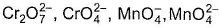,Cu2O and Ni-DMG complex are coloured.

Statement II : It is due to charge transfer transition.

Solution:

Colours of,Cu2O and Ni-DMG complex are due to charge transfer transition.
(iv) Sodium sulphite is a reducing agent. It reduces acidified K2CrO7 to chromic sulphate which is green in colour.